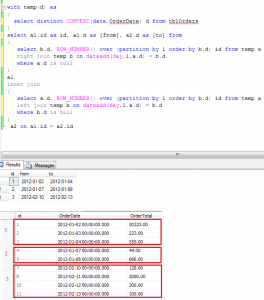# Finding non empty data periods T-SQL

Ever wanted to find non empty periods of data using T-SQL? I this article I will show you how to do it.
Example below shows the periods of days in which there have been at least one order. The days without orders are not being shown. This is very useful when creating advanced sales reports. Having non empty periods we can calculate additional values such as average sales in these ranges etc.

The code below simply gets all distinct dates then creates two partitions and joins them together.
In each partition the data are joined with each other using left and right join. The non null values are next being used in the final inner join.

``` with temp(d) as
(
select distinct CONVERT(date,OrderDate) d from tblOrders
)
select a1.id as id, a1.d as [from], a2.d as [to] from
(
select b.d, ROW_NUMBER() over (partition by 1 order by b.d) id from temp a
right join temp b on dateadd(day,1,a.d) = b.d
where a.d is null
)
a1
inner join
(
select a.d, ROW_NUMBER() over (partition by 1 order by b.d) id from temp a
left join temp b on dateadd(day,1,a.d) = b.d
where b.d is null
)
a2 on a1.id = a2.id
```In order to calculate sales data in periods, simply join the final results using found dates. Hope I helped you with this sample. Happy coding 🙂(No Ratings Yet)Loading...
Tags: